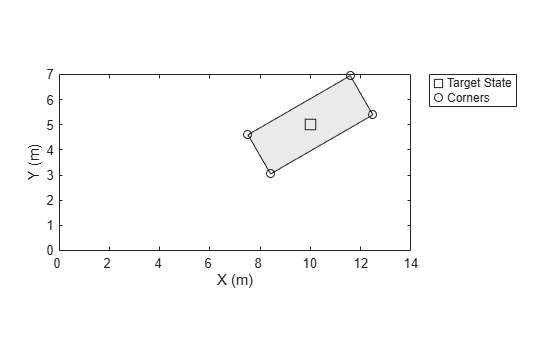Main Content

# ctrectcorners

Corner measurements of constant turn-rate rectangular target

## Syntax

``zCorners = ctrectcorners(states)``
``zCorners = ctrectcorners(states,sensorParameters)``

## Description

````zCorners = ctrectcorners(states)` returns the positions of the corners for constant turn-rate rectangular targets in a rectangular frame. ```

example

````zCorners = ctrectcorners(states,sensorParameters)` specifies the parameters of the sensor that measures the corners of rectangular targets. ```

## Examples

collapse all

Define sensor reference frame by specifying the `sensorParameters` input.

```sensorPosition = [-5;10;0]; sensorOrientation = rotmat(quaternion([30 0 0],'eulerd','ZYX','frame'),'frame'); sensorParams = struct('Frame','Rectangular', ... 'OriginPosition',sensorPosition,... 'Orientation',sensorOrientation);```

Define the constant turn-rate state for the rectangle target.

`state = [10;5;1.6;30;0.5;4.7;1.8];`

Compute corner positions in sensor reference frame.

`corners = ctrectcorners(state,sensorParams);`

Set up visualization environment using `theaterPlot`.

```% Create a theater plot. tp = theaterPlot; % Plot the state using a track plotter. statePlotter = trackPlotter(tp,'DisplayName','Target State'); % Plot the corners using a detection plotter. cornerPlotter = detectionPlotter(tp,'DisplayName','Corners');```

Compute inputs and plot.

```targetPos = [state(1) state(2) 0]; targetOrientation = rotmat(quaternion([state(4) 0 0],'eulerd','ZYX','frame'),'frame'); targetDims = struct('Length',state(6),... 'Width',state(7),... 'Height',5,... 'OriginOffset',[0 0 0]); cornerPosGlobal = sensorOrientation*corners(:,:) + sensorPosition; statePlotter.plotTrack(targetPos,targetDims,targetOrientation); cornerPlotter.plotDetection(cornerPosGlobal');```## Input Arguments

collapse all

Current rectangular target states, specified as a 7-by-N real-valued matrix, where N is the number of states. The seven dimensional rectangular state is defined as [x; y; s; θ; ω; L; W]. The meaning of these variables and their units are:

 Variable Meaning Unit x Position of the rectangle center in x direction m y Position of the rectangle center in y direction m s Speed in the heading direction m/s θ Orientation angle of the rectangle with respect to x direction degree ω Turn-rate degree/s L Length of the rectangle m W Width of the rectangle mExample: `[1;2;2;30;1;4.7;1.8]`

Data Types: `single` | `double`

Parameters for the sensor transform function, returned as a structure or an array of structures. If you only need to transform the state once, specify it as a structure. If you need to transform the state n times, specify it as an n-by-1 array of structures. For example, to transform a state from the scenario frame to the sensor frame, you usually need to first transform the state from the scenario rectangular frame to the platform rectangular frame, and then transform the state from the platform rectangular frame to the sensor spherical frame.

The fields of the structure are:

 Field Description `Frame` Child coordinate frame type, specified as `'Rectangular'` or `'Spherical'`. `OriginPosition` Child frame origin position expressed in the parent frame, specified as a 3-by-1 vector. `OriginVelocity` Child frame origin velocity expressed in the parent frame, specified as a 3-by-1 vector. `Orientation` Relative orientation between frames, specified as a 3-by-3 rotation matrix. If the `IsParentToChild` property is set to `false`, then specify `Orientation` as the rotation from the child frame to the parent frame. If the `IsParentToChild` property is set to `true`, then specify `Orientation` as the rotation from the parent frame to the child frame. `IsParentToChild` Flag to indicate the direction of rotation between parent and child frame, specified as `true` or `false`. The default is `false`. See description of the `Orientation` field for details. `HasAzimuth` Indicates whether outputs contain azimuth components, specified as `true` or `false`. `HasElevation` Indicates whether outputs contain elevation components, specified as `true` or `false`. `HasRange` Indicates whether outputs contain range components, specified as `true` or `false`. `HasVelocity` Indicates whether outputs contain velocity components, specified as `true` or `false`.

Note that here the scenario frame is the parent frame of the platform frame, and the platform frame is the parent frame of the sensor frame.

When `frame` is `'Rectangular'`, `HasVelocity` determines if the measurement is returned in the form of [x; y; z; vx; vy; vz] or [x; y; z].

When `frame` is `'spherical'`, the returned measurements are in the order of [azimuth, elevation, range, range-rate]. The elements of the returned measurements are determined by:

• `HasAzimuth` — Determines if output contains azimuth measurement.

• `HasElevation` — Determines if output contains elevation measurement.

• `HasRange` — Determines if output contains range measurement.

• `HasVelocity` — Determines if output contains range-rate measurement on the condition that `HasRange` is `'true'`. If `HasRange` is `'false'`, the returned measurement does not contain range-rate (even though `HasVelocity` is `'true'`).

Data Types: `struct`

## Output Arguments

collapse all

States of corners, returned as a real-valued M-by-N-by-4 array. Each page (an M-by-N matrix) of the array corresponds to one corner for all the states given in the states input. N is the number of states. M is the dimension of output specified by the `sensorParameters` input. If unspecified, the default value of M is three, which corresponds to 3-D Cartesian position coordinates.

## See Also

Introduced in R2018b

## Support

#### Sensor Fusion and Tracking for Autonomous Systems

Download white paper